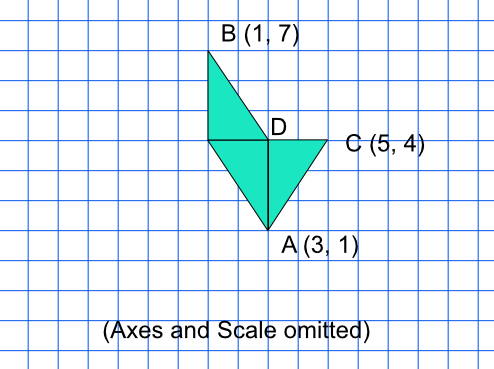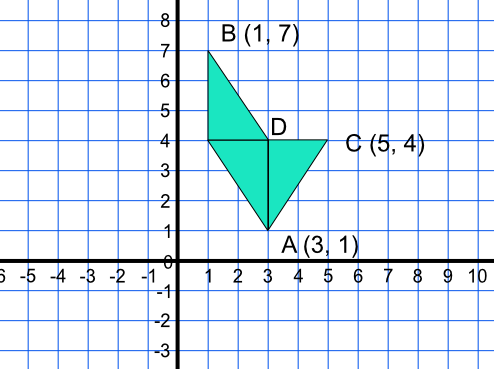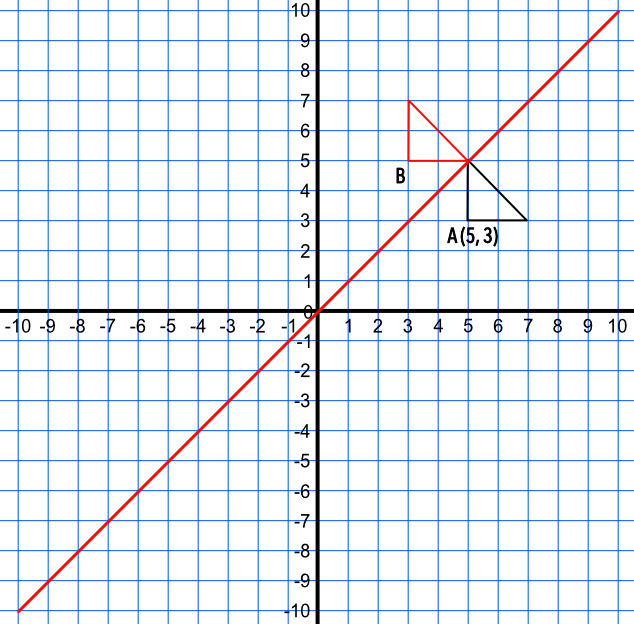Solving Geometry Problems on Axes

## Solving Geometry Problems on Axes

Problems involving coordinates on axes need to be related to the (x, y) coordinates of the axes. Draw or plot any given lines or coordinates. Missing coordinates can be worked out from knowledge of the shape being plotted.

Answers should be given as coordinates (unless otherwise specified).

## Example 1

Three congruent triangles are shown below. What are the coordinates of point D?The triangles are congruent and are therefore all the same size.

The height of two triangles is given by the y-coordinate of B minus the y-coordinate of A

7 - 1 = 6

The height of one triangle = 6 ÷ 2 = 3

Point D is immediately above point A, so the x-coordinate is unchanged.

Point D = (3, 1 + 3) = (3, 4)A triangle has a right angle at point A. The triangle is reflected in the line x=y. What are the coordinates of point B?Draw the reflection in x=y then reflect the triangle.